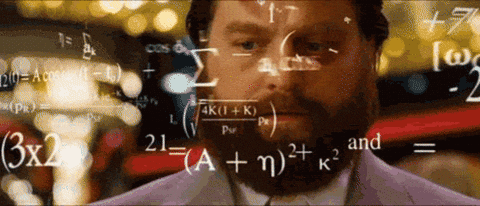## Nonparametric neighbours: U-statistic structural components and jackknife pseudo-observations for the AUC

Two of my recent blog posts focused on two different, but as we will see related, methods which essentially transform observed responses into a summary of their contribution to an estimate: structural components resulting from Sen’s (1960) decomposition of U-statistics and pseudo-observations resulting from application of the leave-one-out jackknife. As I note in this comment, I think the real value of deconstructing estimators in this way results from the use of these quantities, which in special (but common) cases are asymptotically uncorrelated and identically distributed, to: (1) simplify otherwise complex variance estimates and construct interval estimates, and (2) apply regression methods to estimators without an existing regression framework.

As discussed by Miller (1974), pseudo-observations may be treated as approximately independent and identically distributed random variables when the quantity of interest is a function of the mean or variance, and more generally, any function of a U-statistic. Several other scenarios where these methods are applicable are also outlined. Many estimators of popular “parameters” can actually be expressed as U-statistics. Thus, these methods are quite broadly applicable. A review of basic U-statistic theory and some common examples, notably the difference in means or the Wilcoxon Mann-Whitney test statistic, can be found within my blog post: One, Two, U: Examples of common one- and two-sample U-statistics.

As an example of use case (1), Delong et al. (1988) used structural components to estimate the variances and covariances of the areas under multiple, correlated receiver operator curves or multiple AUCs. Hanley and Hajian-Tilaki (1997) later referred to the methods of Delong et al. (1988) as “the cleanest and most elegant approach to variances and covariances of AUCs.” As an example of use case (2), Andersen & Pohar Perme (2010) provide a thorough summary of how pseudo-observations can be used to construct regression models for important survival parameters like survival at a single time point and the restricted mean survival time.

Now, structural components are restricted to U-statistics while pseudo-observations may be used more generally, as discussed. But, if we construct pseudo-observations for U-statistics, one of several “valid” scenarios, what is the relationship between these two quantities? Hanley and Hajian-Tilaki (1997) provide a lovely discussion of the equivalence of these two methods when applied to the area under the receiver operating characteristic curve or simply the AUC. This blog post follows their discussion, providing concrete examples of computing structural components and pseudo-observations using R, and demonstrating their equivalence in this special case.

## Resampling, the jackknife, and pseudo-observations

Resampling methods approximate the sampling distribution of a statistic or estimator. In essence, a sample taken from the population is treated as a population itself. A large number of new samples, or resamples, are taken from this “new population”, commonly with replacement, and within each of these resamples, the estimate of interest is re-obtained. A large number of these estimate replicates can then be used to construct the empirical sampling distribution from which confidence intervals, bias, and variance may be estimated. These methods are particularly advantageous for statistics or estimators for which no standard methods apply or are difficult to derive.

The jackknife is a popular resampling method, first introduced by Quenouille in 1949 as a method of bias estimation. In 1958, jackknifing was both named by Tukey and expanded to include variance estimation. A jackknife is a multipurpose tool, similar to a swiss army knife, that can get its user out of tricky situations. Efron later developed the arguably most popular resampling method, the bootstrap, in 1979 after being inspired by the jackknife.

Good simple ideas, of which the jackknife is a prime example, are our most precious intellectual commodity, so there is no need to apologize for the easy mathematical level.

Despite existing since the 1940’s, resampling methods were infeasible due to the computational power required to perform resampling and recalculate estimates many times. With today’s computing power, the uncomplicated yet powerful jackknife, and resampling methods more generally, should be a tool in every analyst’s toolbox.

## Simplifying U-statistic variance estimation with Sen’s structural components

Sen (1960) proved that U-statistics could be decomposed into identically distributed and asymptotically uncorrelated “structural components.”

The mean of these structural components is equivalent to the U-statistic and the variance of the structural components can be used to estimate the variance of the U-statistic, bypassing the need for often challenging derivation of conditional variance components.

## One, Two, U: Examples of common one- and two-sample U-statistics

My previous two blog posts revolved around derivation of the limiting distribution of U-statistics for one sample and multiple independent samples.

For derivation of the limiting distribution of a U-statistic for a single sample, check out Getting to know U: the asymptotic distribution of a single U-statistic.

For derivation of the limiting distribution of a U-statistic for multiple independent samples, check out Much Two U About Nothing: Extension of U-statistics to multiple independent samples.

The notation within these derivations can get quite complicated and it may be a bit unclear as to how to actually derive components of the limiting distribution.In this blog post, I provide two examples of both common one-sample U-statistics (Variance, Kendall’s Tau) and two-sample U-statistics (Difference of two means, Wilcoxon Mann-Whitney rank-sum statistic) and derive their limiting distribution using our previously developed theory.